# Olympiad Test: Number Ranking - 1

## 15 Questions MCQ Test Science Olympiad Class 8 | Olympiad Test: Number Ranking - 1

Description
Attempt Olympiad Test: Number Ranking - 1 | 15 questions in 30 minutes | Mock test for Class 8 preparation | Free important questions MCQ to study Science Olympiad Class 8 for Class 8 Exam | Download free PDF with solutions
QUESTION: 1

### How many odd numbers are there in the sequence each of which is immediately followed by an odd number? 5 1 4 7 3 9 8 5 7 2 6 3 1 5 8 6 3 8 5 2 2 4 3 4 9 6

Solution: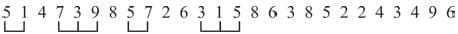QUESTION: 2

### How many odd numbers are there in the Sequence which are immediately preceded and also immediately followed by an even number in the sequence ? 5 1 4 7 3 2 5 6 8 9 6 7 3 2 1 5 6 4 3 2 7 4

Solution: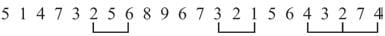QUESTION: 3

### How many even numbers are there which are immediately followed by an odd number and also immediately preceded by an odd number in the sequence? 8 4 7 6 5 3 2 5 1 6 4 3 2 6 7 9 8 5

Solution:QUESTION: 4

In the series
2 5 3 4 8 7 4 2 6 7 1 5 8 3 7 4 5 3
How many pair of successive numbers have a difference of 3?

Solution: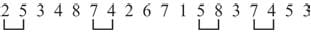QUESTION: 5

How many 5’s are there in the sequence which are immediately preceded by 4 and immediately followed by 7?
2 3 4 5 6 1 4 5 7 1 2 4 5 7 3 8 4 5 9 6 5 3

Solution: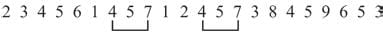QUESTION: 6

How many pair of successive numbers are in the given series whose sum is 12?
4 5 7 3 2 8 4 9 1 5 6 4 8 3 1 9 3 5 7

Solution: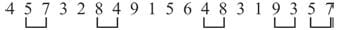QUESTION: 7

How many pair of successive numbers ar ein the given series whose product is an even number?
6 1 4 6 3 5 1 7 2 6 3 5 7 1 8 3 7 5

Solution: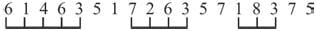QUESTION: 8

How many 9’s are there in the series which are preceded by 7 but not followed by 8?
4 2 9 6 7 9 2 4 7 9 8 4 7 9 3 6 8 9

Solution: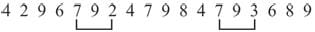QUESTION: 9

What is the product of sum of even digits and sum of odd digits in the number
8 6 7 4 3 5 2?

Solution:

Sum of even digits = 8 + 6 + 4 + 2 = 20
Sum of odd digits = 7 + 3 + 5 = 15
Product = 20 × 15 = 300

QUESTION: 10

How many 8’s are there in the series which are preceded by 5 but not followed by 7?
4 3 8 6 5 8 2 3 5 8 4 7 5 8 7 4

Solution: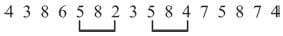QUESTION: 11

What is the difference between the sum of even digits and sum of odd digits in the number
7 4 6 2 5 8 9 3?

Solution:

Sum of even digits = 4 + 6 + 2 + 8 =20
Sum of odd digits = 7 + 5 + 9 + 3 = 24
Difference = 24 – 20 = 4.

QUESTION: 12

If the given series is written in the reverse order which number will be 5th to the right of the seventh number from the left?
7, 3, 8, 7, 0, 3, 4, 6, 2, 5, 9, 6, 4, 7

Solution:

Given series 7, 3, 8, 7, 0, 3, 4, 6, 2, 5 , 9, 6, 4, 7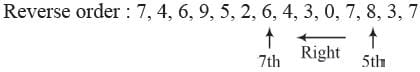QUESTION: 13

In the given number sequence, how many such even numbers which are exactly divisible by its immediate preceding number but not exactly divisible by its immediate succeeding number?
3 5 6 4 8 7 1 2 4 3 6 4 5 2 6 7

Solution: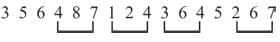QUESTION: 14

From the given numbers
823, 716, 539, 683, 937
what will be the first digit of the second highest number after the position of only second and third digits with in each number are interchanged ?

Solution:

Given numbers – 823, 716, 539, 683, 937
New numbers – 832, 761, 593, 638, 973
Second highest number = 832.
Ist first digit = 8

QUESTION: 15

From the given numbers
728, 593, 645, 296, 968
what will be the first digit of the second highest number after the position of only first and third digits within each number are
interchanged?

Solution:

Given numbers – 728, 593, 645, 296, 968
New numbers – 827, 395, 546, 692, 869
Second highest number = 827
Ist first digit = 8Use Code STAYHOME200 and get INR 200 additional OFF Use Coupon Code